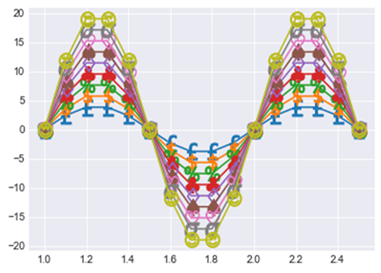# How to create custom markers on a plot in Matplotlib

To create a custom marker on a plot or graph, we use a list where we write the markers we want to see in the plot. The markers are nothing but symbols, emoji, character or any character which we want to see on the figure.

In order to create the marker, we will first import the required libraries.

import matplotlib.pyplot as plt
import numpy as np

For now, we will create a marker on a sine curve. Let us create the grid with size (12,6),

x = np.arange(1, 2.6, 0.1)
y = np.sin(2 * np.pi * x)
plt.subplots(figsize=(12,6))

Here we will create the list of custom markers,

custom_markers = ['$'+x+'$' for x in
['£','\\$','\%','\clubsuit','\diamondsuit','\spadesuit','\heartsuit','\sigma','😍" />']]

Now let us plot the sine Curve,

for i,marker in enumerate(custom_markers):
plt.plot(x, 2*(i+2)*y, marker=marker, markersize=15)

Display the figure,

plt.show()

## Output